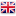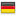# Sinergit Ltd.You are here:
13 August 2022

### vertical application - Massa in free fall(W) Mass = 2500 kg
(H) Height = 2 m

C = 9.81 * W * H
And c * = 9.81 2500 2 *

NB. In the case where the weight remains bearing on the bumper, the arrow of the latter must not exceed 30% of its length.

### Horizontal application - live load(W) Mass = 50000 kg
(V) Speed ​​= 20 m / min = 20/60 = 0.33 m / s

C = 1 / 2 2 * W * V ^
And c * = 0.5 50000 0.33 * ^ = 2 2722.5 Nm

### Application with load falling on an inclined plane(W) Mass = 1000 kg
(H) Height = 2 m
(à) Angle of inclination = 30 °

C = 9.81 * W * H
C = 9.81 1000 * * = 2 19620 Nm

NB. If the mass remains on the bumper, the deflection of the latter must not exceed 30% of its length.

### Horizontal application - Load into rotary motion(W) Mass = 2500 kg
(⩊) Angular velocity = 2 rad / sec
(R) Rotation radius = 0.5 m

C = I * ^ ⩊ 2 / 2
I = W * R ^ 2
* I = 2500 0.5 2 ^
I = 625 2 Nm sec ^
C = 625 2 * ^ 2 / 2
C = 1250 Nm

### Application with opposing bumpersIn this case to proceed to the choice of the bumper, being the energy absorbed by half by each of the two buffers, it must be considered as the value of the mass half the value actually borne on the movable bumper.